Time Travel Research Center © 2005 Cetin BAL - GSM:+90  05366063183 - Turkey / Denizli

 To the Stars: The Reality of Warpdrive Physics- Sun Feb 1 21:38:10 2004 GMT

bludot  writes:

# Introduction

I'm sure many people have wondered about the feasibility of travelling to other stars. In this article I hope to give a semi-quantitive overview of some of the current research in to warp drives and hopefully inspire some of you to take a further interest in what I consider be quite an interesting topic.

I will not give a full definition of a warp drive right now since we haven't done the necessary mathematics. However, a qualitive description of the properties that we require of a warp drive is useful since we then have something to aim for. So, what properties are we looking for? Ideally we would like a way by which a human could travel interstellar distances and return within a single lifetime of both those on board the ship and those that remain on the Earth.

# A Short Introduction to General Relativity

General Relativity was the theory proposed by Einstein in 1915 that incorporated gravity into his special theory of relativity. I won't go into the details of how he did this or explain why Newton's theory of gravity was incompatible with SR here, but I will use some results from General Relativity and they will be explained when they are called upon.

## Length

Of central importance to our discussion is the concept of length and it's more generalised counterpart, an interval (you can think of the interval as a length that includes time). The reason for it's importance originates from the fact that it is a physically invariant quantity, hence all observers will measure the same interval between events. This sort of invariance is most easily visualised in terms of the result of some experiment; for instance the outcome of measuring the length of a rod in normal everyday space is not dependent on the nature of the coordinate system we impose on space. The length of the rod is a physical reality distinct from any arbitrary choice we may make. We say length is invariant under rotations in 3D space. At least in Euclidean (flat) space ;)

### Newtonian Length

We have briefly referred to our natural concept of length previously, we now will discuss it in more detail. In Newtonian mechanics the concept of an interval does not really exist. Newtonian mechanics makes a distinction between spatial and temporal separation, i.e. separation in space and separation in time of events. The spatial separation of two events we call length and the temporal we call time. Furthermore, we have a formula for calculating length, the well known Pythagoras' Theorem, which if we are using a Cartesian coordinate system, has the form: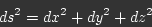However, let us change to a cylindrical coordinate system, the formula for length then becomes,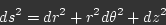Or if we change to spherical coordinates the formula for length becomes,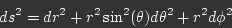So it is clear that the formula for length is certainly dependent on the coordinate system we choose. We could write this more generally as,where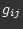represents the coefficient of a term in the formula for. We can expressin the form of a matrix, so for the case of Cartesian coordinates,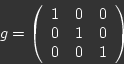(1)

This is actually a mathematical object known as the metric tensor, and encodes how length is calculated. Now that we have developed a slightly more sophisticated understanding of length, we will move on to Special relativity and how the definition of length changes to accommodate this theory.